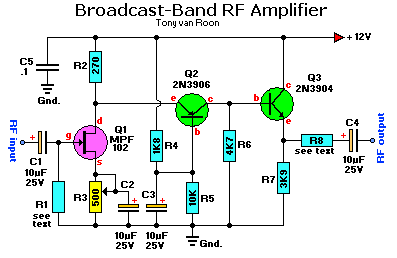By Tony van RoonHow it Works:
The complete schematic is shown in Fig. 1. The circuit has frequency response ranging from 100Hz to 3MHz; gain is about 30dB.
Field Effect Transistor Q1 is configured in the common-source self-biased mode; optional resistor R1 allows you to set the input impedance to any desired value. Commonly, it will be 50 ohms.
This signal is he direct-coupled to Q2, a common-base circuit that isolates the input and output stages and provides the amplifier's exceptional stability.
Last, Q3 functions as an emitter-follower, to provide low output impedance (about 50 ohms). If you need higher output impedance, include resistor R8. It will affect impedance according to this formula: R8 ~ Rout - 50. Otherwise, connect output capacitor C4 directly to the emitter of Q3.

Construction:
The circuit can be wired up on a piece of perfboard; a PC board is not necessary, although one can be used. However you build the circuit, keep lead lengths short and direct, and separate the input and output stages. You may have to install the amplifier in your receiver. Otherwise, installing it in a metal case will reduce stray-signal pickup. You'll have to provide appropriate connectors on the case. Connect the amplifier to the antenna and radio using short lengths of coax.
The circuit has only one adjustment. Connect a source of 12-volt DC power to the circuit, and adjust R3 so that there is a 1.6-volt drop across R2.
If you're not sure of the impedance of your antenna, connect a 500-ohm potentiometer for R1, and adjust it for best reception. Then substitute a fixed-value resistor for the potentiometer.
You may want to follow the same procedure with the output circuit (R8), if you're not sure of your receiver's input impedance. Common impedances are 50, 75, and 300 ohms, so the same 500-ohm potentiometer can be used.
You can connect an external antenna through the amplifier to a receiver that has only a ferrite rod antenna. Connect the amplifier's output to a coil composed of 10-15 turns of #30 hookup wire wound around the existing ferrite core, near the existing winding. To obtain best reception, experiment with the number of turns and their placement. You may need to reverse the connection to the coil if the output is weak.

```Parts List:
R1 = see text                C1,C2,C3,C4 = 10uF, 25volt electrolytic
R2 = 270 ohms                         C5 = 0.1uF, ceramic disc
R3 = 500 ohm potentiometer
R4 = 1K8 (1800 ohms)                  Q1 = MPF102 J-FET, or use NTE451
R5 = 10K (10,000 ohms)                Q2 = 2N3906, PNP-transistor (or use NTE159)
R6 = 4K7 (4700 ohms)                  Q3 = 2N3904, NPN-transistor (or use NTE123AP)
R7 = 3K9 (3900 ohms)                       see note about the 'AP' extension.
R8 = see text
```
Back to Circuits page